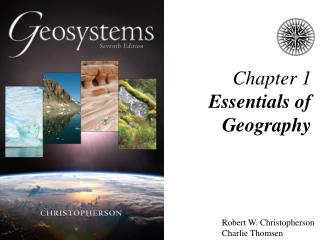DownloadDownload PresentationChapter 1 Essentials of Geography

# Chapter 1 Essentials of Geography

Télécharger la présentation## Chapter 1 Essentials of Geography

- - - - - - - - - - - - - - - - - - - - - - - - - - - E N D - - - - - - - - - - - - - - - - - - - - - - - - - - -
##### Presentation Transcript

1. Chapter 1Essentials of Geography Robert W. Christopherson Charlie Thomsen

2. Essentials of Geography • The Science of Geography   • Earth Systems Concepts   • Location and Time on Earth   • Maps, Scales, and Projections   • Remote Sensing and GIS

3. The Science of Geography • Geography – from geo “Earth” and graphein “to write”  • Geography is • a method, not a body of knowledge • holistic • eclectic • Geographers use spatial analysis • Geographers use Earth systems science

4. Geography Is • The science that studies the relationships among • natural systems • geographic areas • society • cultural activities • and the interdependence of all of these over space

5. Physical Geography Is • The spatial analysis of all the physical elements and processes that make up the environment

6. Figure 1.2

7. Scientific Method Figure FS 1.1.1

8. Earth Systems Concepts • Systems theory   • Open systems • Closed systems • System feedback • System equilibrium

9. Figure 1.3

10. Systems Figure 1.4

11. Systems in Geosystems Figure 1.7

12. Earth’s Four Spheres Figure 1.8

13. Positive FeedbackinHigh-Latitude Meltponds Figure HLC 1.1.1

14. Earth’s Dimensions Figure 1.9

15. Measuring Earth in 247 B.C. Figure 1.10

16. Location and Time on Earth   • Latitude • Longitude • Great circles • Prime Meridian and standard time

17. Latitude Figure 1.11

18. Latitudinal Geographic Zones Figure 1.12

19. Longitude Figure 1.13

20. Great Circles and Small Circles Figure 1.14

21. Prime Meridian and Standard Time  Figure 1.17

22. Maps, Scales, and Projections   • Map – a generalized view of an area, as seen from above and reduced in size • Scale – ratio of map units to ground units • Projection – process of transforming spherical Earth to flat map

23. Classes of Map Projections Figure 1.21

24. Remote Sensing • Active remote sensing • Passive remote sensing

25. Active and Passive Remote Sensing Figure 1.25

26. Remote Sensing RADARSAT-1 GOES-12 Figure 1.26 Figure 1.27

27. Geographic Information Systems (GIS)  • GIS systems combine spatial and attribute data  • Maps can contain multiple data layers: • Physical features • Cultural features • Layers can be added to create composite overlay

28. GIS System Model Figure 1.29

29. End of Chapter 1 Geosystems 7e An Introduction to Physical Geography